CCP4 study weekendBIOLOGICALCRYSTALLOGRAPHY
ISSN: 1399-0047
Volume 59| Part 11| November 2003| Pages 1974-1977

On symmetries of substructures

aLawrence Berkeley National Laboratory, One Cyclotron Road, BLDG 4R0230, Berkeley, California 94720-8235, USA
*Correspondence e-mail: rwgrosse-kunstleve@lbl.gov

(Received 1 May 2003; accepted 24 September 2003)

This paper accompanies a lecture given at the 2003 CCP4 Study Weekend on experimental phasing. With the audience of the CCP4 Study Weekend in mind, an overview is given of symmetries of substructures and the implications for single isomorphous replacement and single anomalous diffraction phasing procedures, as well as difference Fourier analyses. Pointers are also provided to practical tools for working with substructure symmetries.

1. Introduction

Experimental phasing divides the larger problem of determining a complete macromolecular structure into two steps. Firstly, a substructure consisting of heavy atoms or anomalous scatterers is determined and refined. The refined substructure is then used in algebraic or probabilistic phasing procedures to derive estimates of the phases for the full structure. These phases are in turn used to compute electron-density maps for the purpose of density modification and model building (e.g. Drenth, 1999).

In this paper, we give an overview of substructure symmetries. We distinguish two types, expected symmetry and unexpected symmetry. Expected symmetry encompasses alternative origin choices, hand ambiguity and indexing ambiguities. Expected symmetry has to be considered when substructures are compared or combined in some way, e.g. in a multiple isomorphous replacement procedure.

Unexpected symmetry refers to cases where the symmetry of the isolated substructure is higher than the crystal symmetry. For example, in many non-centrosymmetric space groups a single-atom substructure is centrosymmetric. This may have an impact on single isomorphous replacement (SIR) or single anomalous diffraction (SAD) experiments, as well as on difference Fourier analyses. Phasing procedures and difference Fourier syntheses will yield maps that exhibit the higher symmetry of the substructure, rendering substructure completion, density modification and model building more difficult. We introduce a simple diagnostic test that indicates unexpected substructure symmetry.

2. Expected symmetry

Expected symmetry is equivalent to or a subgroup of the Euclidean normalizer of the crystal symmetry (Koch & Fischer, 1983), which is also known as Cheshire symmetry (Hirshfeld, 1968). The concepts of allowed origin shifts or structure-seminvariant vectors and moduli (e.g. Giacovazzo, 2001) are also intimately connected with Euclidean normalizers. The exact definition of Euclidean normalizers as given by, for example, Koch & Fischer (1983), is an advanced subject of group theory and a full account is beyond the scope of this paper. Here, we will approach Euclidean normalizers pragmatically by noticing that they are useful for answering the question How many possibilities are there for transforming a given (sub)structure without changing the corresponding diffraction intensities?'

We can distinguish three classes of transformations, corresponding to the three columns listed under Additional Generators' in Table 15.3.2 of Hahn (1983).

2.1. Translations

A simple example is space group P1 (No. 1). The only symmetry is the periodicity of the lattice of unit cells. If a given substructure is shifted arbitrarily in space, the complex structure factors change owing to phase shifts, but the intensities are invariant. Fig. 1illustrates that this is not the case for (for example) space group P2 (No. 3). An atom placed at the site xyz implies another atom at −xy, −z. If both atoms are shifted together in a general direction and the symmetry is applied again, the result is that we find four atoms in the unit cell. The overall arrangement of atoms is invariant only for particular shifts (Fig. 1c). These are known as allowed origin shifts, which can alternatively be represented as structure-seminvariant vectors and moduli (e.g. Giacovazzo, 2001).Figure 1 Allowed origin shifts. (a) An atom in P2 and its symmetrically equivalent copy in four unit cells. (b) The two atoms arbitrarily shifted and their symmetrically equivalent copies. The overall structure changes under crystal symmetry. (c) The two atoms shifted by the allowed origin shift ½, ½, 0. The overall structure is invariant under crystal symmetry.

2.2. Inversion through a center

In the absence of anomalous scattering, the inverse image of a given structure gives rise to the same diffraction intensities as the original structure. Somewhat counter-intuitively, this is also true for substructures solved using anomalous differences, as these are approximations of the anomalous contributions to the structure factor only (e.g. Grosse-Kunstleve & Adams, 2003a). In most space groups the inversion operation is located at the origin of the standard setting, but there are exceptions (e.g. space group I4122; No. 98). Applying the inversion operation is also known as changing the hand' of a structure.

2.3. Further generators

These generators describe the possibilities for reindexing a given data set. For some space groups, autoindexing programs may randomly choose between alternative indexing conventions (c.f. Scenario 5: Reindexing' in the SCALEPACK Manual published by HKL Research Inc.; http://www.hkl-xray.com/hkl/manual.htm ). For example, in P4 a certain reflection could be indexed as (1, 2, 0) or alternatively (2, 1, 0). This corresponds to the generator yxz in Table 15.3.2 of Hahn (1983).

To compare two substructures that were derived from the same data set, it is sufficient to consider the allowed origin shifts and the inversion through a center as given by Table 15.3.2 of Hahn (1983). If it is not a given that the substructures relate to the same data set, the further generators must also be taken into account. This is performed automatically by the Euclidean model-matching (Emma) module included in the Computational Crystallography Toolbox (Grosse-Kunstleve & Adams, 2003b). Similar programs are included in the Shake-and-Bake suite (Smith, 2002) and the SHELX suite (Dall'Antonia et al., 2003).

3. Unexpected symmetry

An electron-density map obtained through SIR phasing is a superposition of the true electron density and the centrosymmetric counterpart of the true electron density convoluted with the Fourier transform of exp(2iφsub), where φsub are the phases of the heavy-atom substructure and i is the imaginary number. The Fourier coefficients of this map are given by (Ramachandran & Srinivasan, 1970)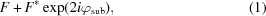where F represents complex structure factors and F* are the complex conjugates.

An electron-density map obtained through SAD phasing is a superposition of the true electron density and the negative inverse of the true electron density convoluted with the Fourier transform of exp(2iφsub) (Ramachandran & Srinivasan, 1970),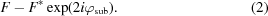The second terms of (1)and (2)are expected to only contribute noise to the general background in the SIR and SAD maps, respectively. However, the case when the substructure has a centrosymmetric configuration in a noncentrosymmetric crystal is special. If the center of inversion of the substructure is (without loss of generality) placed at the origin of the unit cell, all phases φsub are either 0 or 180° and exp(2iφsub) = 1 for either value. Therefore, (1)and (2)reduce to F + F* and F − F*, respectively. The SIR or SAD map will therefore be the superposition of the true electron density with its exact inverse or exact negative inverse, respectively, and interpretation of the map can be significantly more difficult.

Often it is not immediately obvious that a substructure is centrosymmetric. However, the following algorithm provides a simple means to test for this condition.

 (i) Given the crystallographic data of a substructure, the phases φsub are computed through a straightforward structure-factor calculation. (ii) The coefficients exp(2iφsub) are Fourier transformed. (iii) The resulting map is searched for peaks. (iv) The peaks are sorted by height and plotted. (v) If the resulting plot shows one sharp peak then the substructure is centrosymmetric.
We refer to this diagnostic procedure as the phase-o-phrenia algorithm (http://cci.lbl.gov/cctbx/phase_o_phrenia.html ). This name is inspired by the term double-phasing' used by Ramachandran & Srinivasan (1970) in reference to SIR and SAD phasing. The rationale behind the phase-o-phrenia algorithm is that the Fourier transform of a constant function [e.g. exp(2iφsub) = 1] is a delta function. It is different from zero only at the origin and exactly zero everywhere else. If the center of inversion of a substructure is shifted away from the origin, the expression exp(2iφsub) is no longer a constant but the Fourier transform is still a delta function which is different from zero only at the location (2xc, 2yc, 2zc), where (xc, yc, zc) are the coordinates of the center of inversion.

Figs. 2, 3, 4and 5show selected results of the phase-o-phrenia algorithm. The sharp peak in Fig. 2is generated by one or two atoms in any position in space group P1 or, for example, one atom at x1, y1, 0 and optionally another atom at x2, y2, ½ in space group P6. As a counter-example, Fig. 3shows a plot generated by four randomly placed atoms in space group P31. The phase-o-phrenia algorithm can also be used to show that some maps will be more difficult to interpret than others even if the substructure is not centrosymmetric. For example, the plot generated by a randomly placed atom in space group P3 (Fig. 4) is significantly sharper than a plot generated by a randomly placed atom in space group P31 (Fig. 5). This reflects the higher symmetry of the single-atom substructure in P3: the symmetry is actually, with a mirror plane passing through the atom.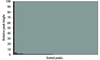Figure 2 Phase-o-phrenia plot for centrosymmetric substructures, e.g. one or two atoms in P1 or one atom at x, y, 0 in P6. The sharp plot reflects that the substructures are centrosymmetric.Figure 3 Phase-o-phrenia plot for four randomly placed atoms in P31. The flat plot indicates that the substructure symmetry is identical to the crystal symmetry.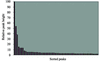Figure 4 Phase-o-phrenia plot for one randomly placed atom in P3. The relatively sharp plot is the result of thesymmetry of the substructure.Figure 5 Phase-o-phrenia plot for one randomly placed atom in P31. The relatively flat plot illustrates the contrast to a single-atom substructure that results in a higher symmetry (Fig. 4).

It is worth noting that substructures with all sites in special positions may also lead to higher symmetries. A systematic treatment can be found in chapter 14 of Hahn (1983) under the title Lattice Complexes'.

4. Implications for difference Fourier analyses

It is possible to solve small substructures by manual difference Fourier analyses. A certain number of initial sites (often only one) are used to compute phases for the full structure. These phases are combined with isomorphous or anomalous differences ΔF to compute a Fourier map that hopefully shows the missing substructure sites,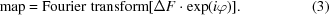If the substructure used to compute the phases φ has a symmetry higher than the crystal symmetry, the phases will reflect this higher symmetry. Difference Fourier maps in both the SIR and SAD case are likely to show spurious strong positive peaks. For example, if the substructure is centrosymmetric, the map will show the substructure and the inverse substructure simultaneously. Therefore, it is important to pick only one peak from the difference Fourier map and to repeat the computation of phases with the additional site. If more than one site is picked from the same map they may not all be consistent with one choice of hand and the phasing procedure could fail. The best way to avoid this pitfall is to use automatic procedures for the solution of substructures (e.g. Grosse-Kunstleve & Adams, 2003a). In our experience, it is highly unlikely that a substructure can be solved manually if all automatic programs available fail to produce the solution.

5. Conclusions

We have presented a summary of how Euclidean normalizer symmetry affects the comparison of substructures found in experimental phasing procedures. Based on this theory, we have implemented a Euclidean model-matching algorithm (Emma; http://cci.lbl.gov/cctbx/emma.html ) that is freely available as source code and through a web interface. Details of the Emma algorithm will be published elsewhere.

We have also shown how substructures with a symmetry higher than the crystal symmetry may affect the interpretability of SIR and SAD maps. Our simple diagnostic phase-o-phrenia algorithm is also freely available as source code and through an easy-to-use web interface. For substructures with a small number of sites (less than five), it can be informative to use the phase-o-phrenia web service. The general rule is that a SIR or SAD map will be easier to interpret if the phase-o-phrenia plot is relatively flat because the (negative) inverse of the electron density is smeared out more evenly according to (1)and (2). A sharp plot indicates that a map may be difficult to interpret even if the diffraction data are of high quality.

Acknowledgements

Our work was funded in part by the US Department of Energy under contract No. DE-AC03-76SF00098 and by NIH/NIGMS under grant No. 1P01GM063210.

ReferencesBIOLOGICALCRYSTALLOGRAPHY